Anzeige

# collections-cake-toppers

jackreay
17. Mar 2023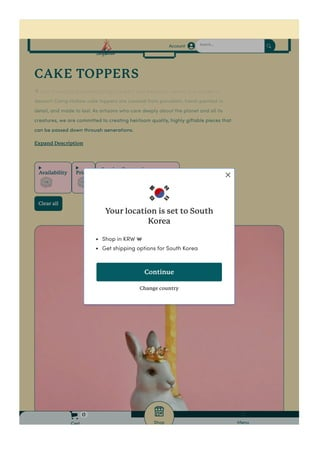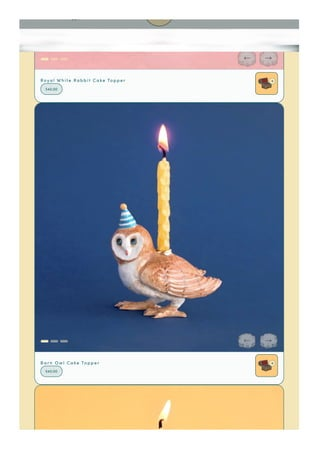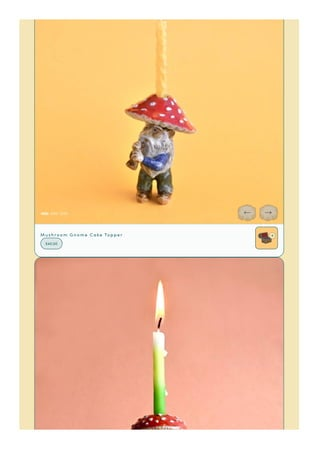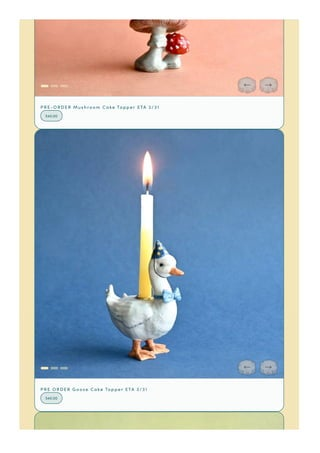Anzeige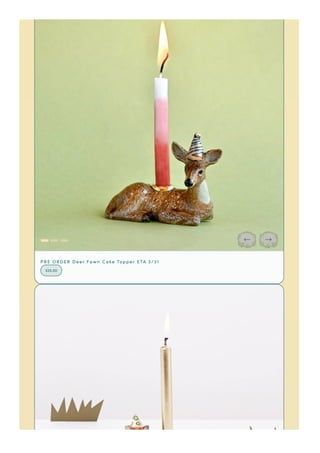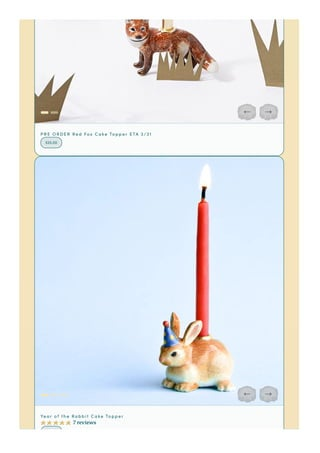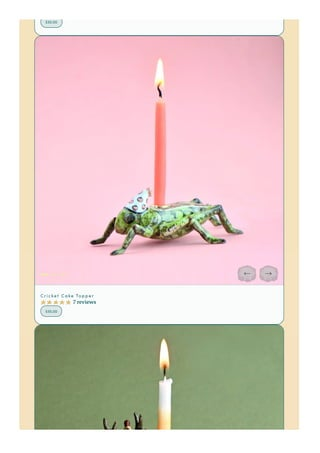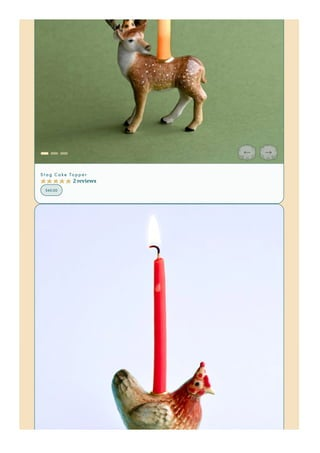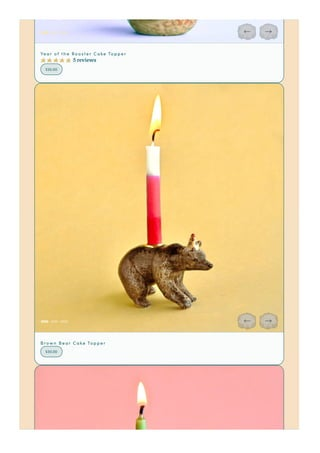Anzeige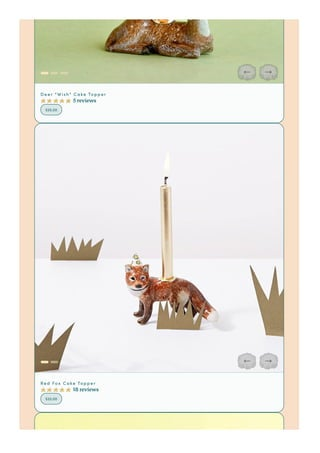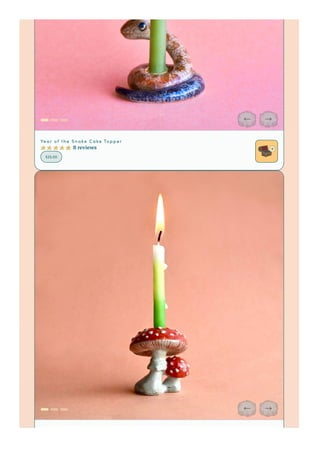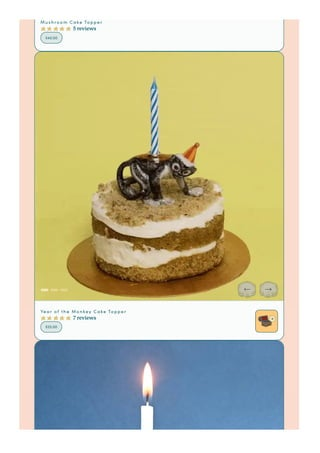Anzeige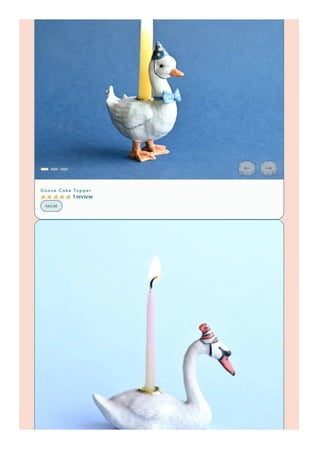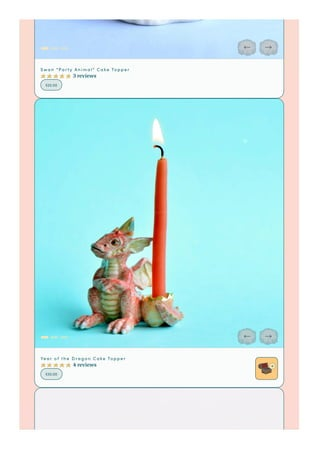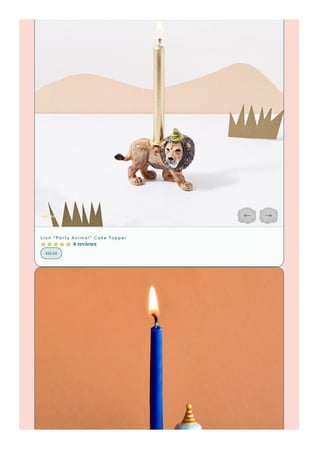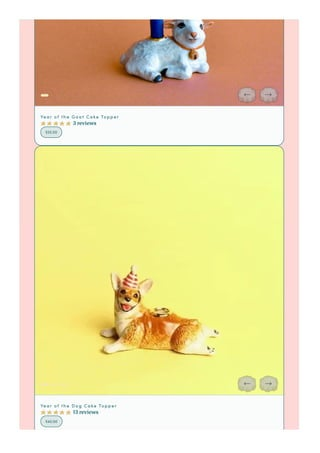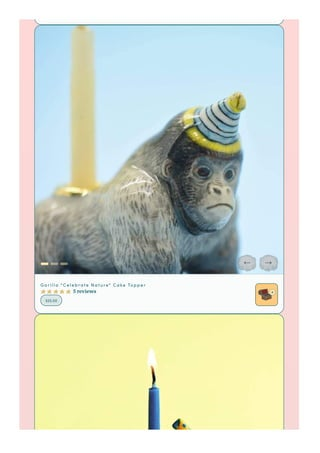Anzeige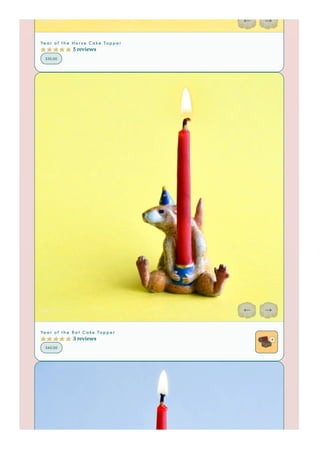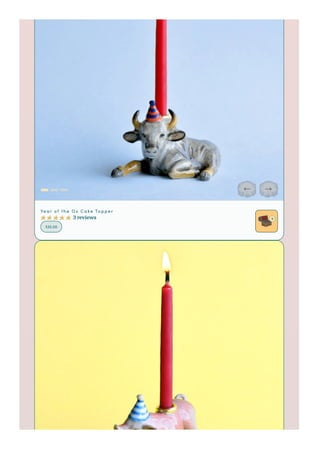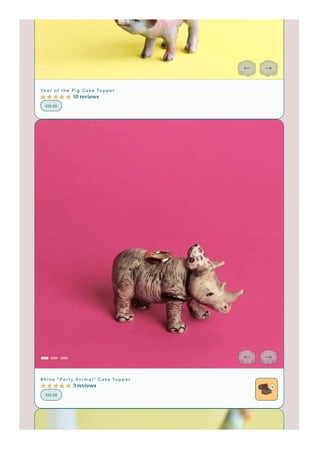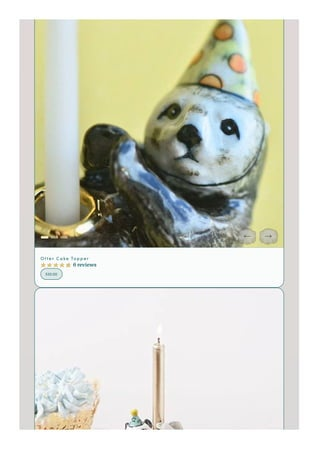Anzeige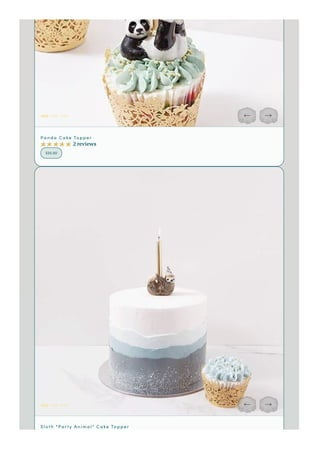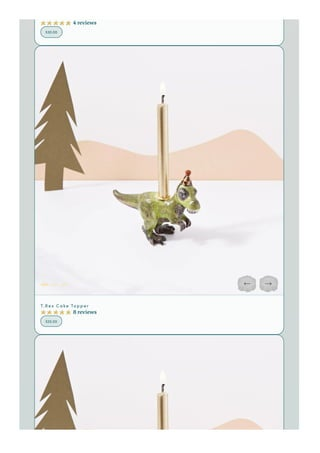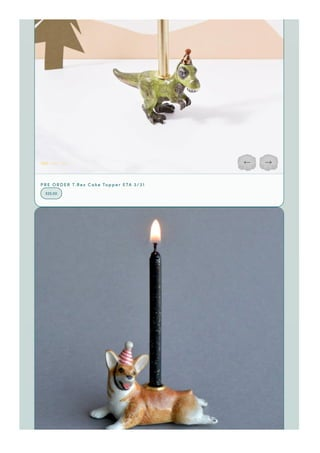Anzeige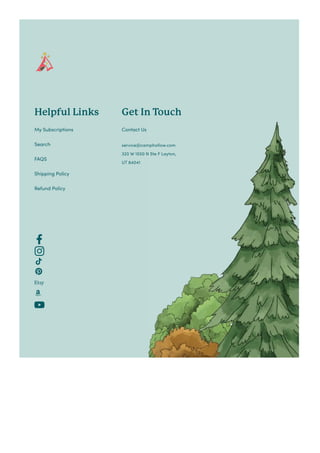Nächste SlideShare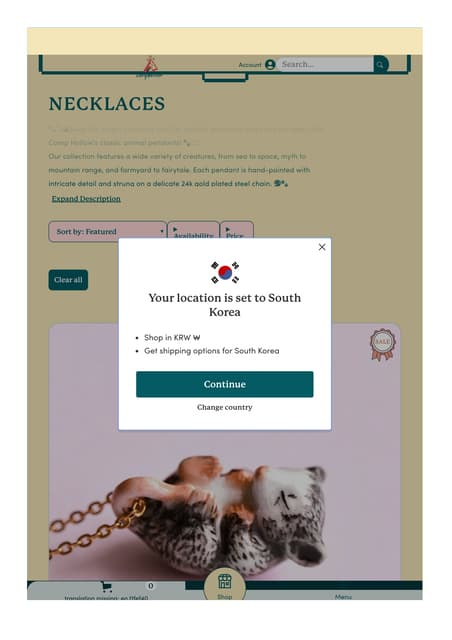camp hollow
1 von 30
Anzeige

### collections-cake-toppers

1. CAKE TOPPERS Expand Description Sort by: Featured Clear all Ἰ Our line of party animals bring the spirit and beauty of nature to any cake or dessert! Camp Hollow cake toppers are created from porcelain, hand-painted in detail, and made to last. As artisans who care deeply about the planet and all its creatures, we are committed to creating heirloom quality, highly giftable pieces that can be passed down through generations. Ἰ Our line of party animals bring the spirit and beauty of nature to any cake or dessert! Camp Hollow cake toppers are created from porcelain, hand-painted in detail, and made to last. As artisans who care deeply about the planet and all its creatures, we are committed to creating heirloom quality, highly giftable pieces that can be passed down through generations. Availability Price Account Search… ç å m p h ø l l ø w Shop Menu Cart 0 Y our location is set to South Korea Shop in KRW ₩ Get shipping options for South Korea Continue Change country
2. R o ya l W h i t e R a b b i t C a k e To p p e r \$40.00 B a r n O w l C a k e To p p e r \$40.00 Shop Menu Cart
3. M u s h r o o m G n o m e C a k e To p p e r \$40.00
4. P R E -O R D E R M u s h r o o m C a k e To p p e r E T A 3/31 \$40.00 P R E O R D E R G o o s e C a k e To p p e r E T A 3/31 \$40.00
5. P R E O R D E R D e e r F a w n C a k e To p p e r E T A 3/31 \$25.00
6. P R E O R D E R R e d F o x C a k e To p p e r E T A 3/31 \$25.00 Ye a r o f t h e R a b b i t C a k e To p p e r 7 reviews \$30.00
7. \$30.00 C r i c k e t C a k e To p p e r 7 reviews \$35.00
8. S t a g C a k e To p p e r 2 reviews \$40.00
9. Ye a r o f t h e R o o s t e r C a k e To p p e r 5 reviews \$30.00 B r o w n B e a r C a k e To p p e r \$30.00
10. Ye a r o f t h e T i g e r C a k e To p p e r 6 reviews \$40.00
11. D e e r "W i s h " C a k e To p p e r 5 reviews \$25.00 R e d F o x C a k e To p p e r 18 reviews \$25.00
12. Z e b r a "P a r t y A n i m a l " C a k e To p p e r 8 reviews \$25.00
13. Ye a r o f t h e S n a k e C a k e To p p e r 8 reviews \$25.00
14. M u s h r o o m C a k e To p p e r 5 reviews \$40.00 Ye a r o f t h e M o n k e y C a k e To p p e r 7 reviews \$25.00
15. G o o s e C a k e To p p e r 1 review \$40.00
16. S w a n "P a r t y A n i m a l " C a k e To p p e r 3 reviews \$25.00 Ye a r o f t h e D r a g o n C a k e To p p e r 4 reviews \$30.00
17. L i o n "P a r t y A n i m a l " C a k e To p p e r 4 reviews \$25.00
18. Ye a r o f t h e G o a t C a k e To p p e r 3 reviews \$25.00 Ye a r o f t h e D o g C a k e To p p e r 13 reviews \$40.00
19. G o r i l l a "C e l e b r a t e N a t u r e " C a k e To p p e r 5 reviews \$25.00
20. F l a m i n g o "P a r t y A n i m a l " C a k e To p p e r 4 reviews \$25.00
21. Ye a r o f t h e H o r s e C a k e To p p e r 5 reviews \$35.00 Ye a r o f t h e R a t C a k e To p p e r 3 reviews \$40.00
22. Ye a r o f t h e O x C a k e To p p e r 3 reviews \$35.00
23. Ye a r o f t h e P i g C a k e To p p e r 10 reviews \$35.00 R h i n o "P a r t y A n i m a l " C a k e To p p e r 3 reviews \$25.00
24. O t t e r C a k e To p p e r 6 reviews \$30.00
25. P a n d a C a k e To p p e r 2 reviews \$25.00 S l o t h "P a r t y A n i m a l " C a k e To p p e r
26. 4 reviews \$30.00 T .R e x C a k e To p p e r 8 reviews \$25.00
27. P R E O R D E R T .R e x C a k e To p p e r E T A 3/31 \$25.00
28. P R E O R D E R Ye a r o f t h e D o g C a k e To p p e r E T A 3/31 \$40.00 P R E O R D E R P a n d a C a k e To p p e r 3/31 \$25.00
29. Fo l l ow u s o n I n s t a g ra m @ c a m ph o l l ows t u d i o ! P R E O R D E R Ye a r o f t h e H o r s e C a k e To p p e r 3/31 \$35.00
30. Helpful Links My Subscriptions Search FAQS Shipping Policy Refund Policy Get In T ouch Contact Us service@camphollow.com 320 W 1550 N Ste F Layton, UT 84041
Anzeige